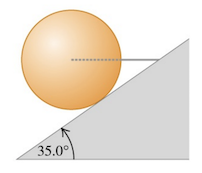# Problem: A horizontal wire holds a solid uniform ball of mass m in place on a tilted ramp that rises 35.0° above the horizontal. The surface of this ramp is perfectly smooth, and the wire is directed away from the center of the ball.(a) Draw a free-body diagram for the ball.(b) How hard does the surface of the ramp push on the ball?(c) What is the tension in the wire?

###### FREE Expert Solution
83% (481 ratings)
###### Problem Details

A horizontal wire holds a solid uniform ball of mass m in place on a tilted ramp that rises 35.0° above the horizontal. The surface of this ramp is perfectly smooth, and the wire is directed away from the center of the ball.
(a) Draw a free-body diagram for the ball.
(b) How hard does the surface of the ramp push on the ball?
(c) What is the tension in the wire?Frequently Asked Questions

What scientific concept do you need to know in order to solve this problem?

Our tutors have indicated that to solve this problem you will need to apply the Equilibrium in 2D concept. You can view video lessons to learn Equilibrium in 2D. Or if you need more Equilibrium in 2D practice, you can also practice Equilibrium in 2D practice problems.

How long does this problem take to solve?

Our expert Physics tutor, Julia took 3 minutes and 45 seconds to solve this problem. You can follow their steps in the video explanation above.

What professor is this problem relevant for?

Based on our data, we think this problem is relevant for Professor Ziffer's class at USM.